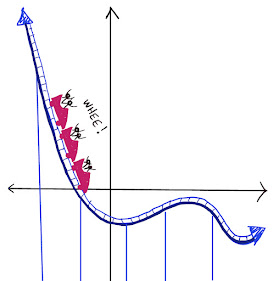## Wednesday, November 24, 2021

### The Stripey Triangle Problem

A triangle is divided into 7 stripes, all the same width and all parallel to the base.  If the small purple triangle at the tip has an area of 3 square units, then what is the area of the largest triangle (the whole thing)?

For the solution, click "Read More" below.

Solution #1:

We can draw in some extra lines and divide the triangle into equal parts.  (It's easiest to see when the triangle is symmetrical (isosceles), but it doesn't have to be.)

The small triangle at the top is one part out of 49 equal parts.  So the whole area is 3 x 49 = 147 square units.

Notice that it is also interesting that counting up the triangles in each stripe gives you the first 7 positive odd integers, which equals  7 x 7:
1 + 3 + 5 + 7 + 9 + 11 + 13 = 49
That is not a coincidence.  In general the sum of the first n positive odd integers is equal to n squared.

Solution #2:
The small purple triangle at the top is similar to the large triangle.  (Since the lines are parallel, the internal angles are equal, and for triangles, that is enough for similarity.)

The side lengths of the triangles are in a ratio of 1 : 7 (because the 7 stripes all have the same width), and therefore their areas are in a ratio of 1 squared : 7 squared, or 1 : 49.
That means if the small triangle has an area of 3, then the large one has an area of 3 x 49:

1 : 49 = 3 : 147.